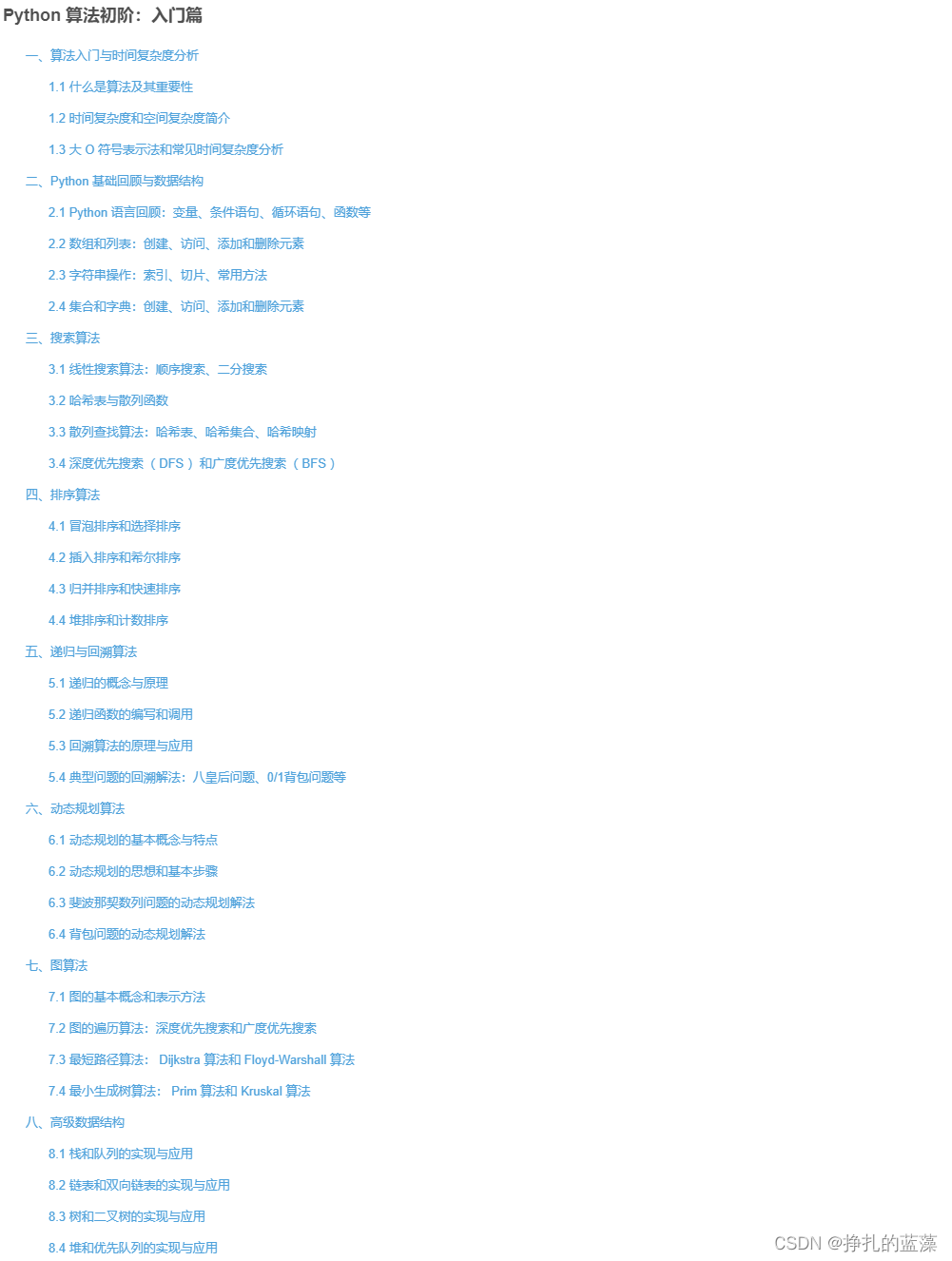# Python 算法基础篇：深度优先搜索（ DFS ）和广度优先搜索（ BFS ）

### Python 算法基础篇：深度优先搜索（ DFS ）和广度优先搜索（ BFS ）

• 引言
• 1. 深度优先搜索（ DFS ）算法概述
• 2. 深度优先搜索（ DFS ）算法实现
• 实例1：图的 DFS 遍历
• 实例2：二叉树的 DFS 遍历
• 3. 广度优先搜索（ BFS ）算法概述
• 4. 广度优先搜索（ BFS ）算法实现
• 实例1：图的 BFS 遍历
• 实例2：二叉树的 BFS 遍历
• 5. DFS 与 BFS 的对比
• 总结

?? ❤️ ❤️ ❤️

## 1. 深度优先搜索（ DFS ）算法概述

DFS 的主要优点是简单且易于实现，它不需要额外的数据结构来记录节点的访问情况，仅使用栈来存储遍历路径。然而， DFS 可能会陷入无限循环中，因为它不考虑节点是否已经访问过。

## 实例1：图的 DFS 遍历

# 图的DFS遍历
def dfs(graph, start, visited):
# 访问当前节点
print(start, end=' ')
# 标记当前节点为已访问
visited[start] = True
# 遍历当前节点的邻居节点
for neighbor in graph[start]:
# 如果邻居节点未被访问，则继续深度优先搜索
if not visited[neighbor]:
dfs(graph, neighbor, visited)

# 图的邻接表表示
graph = {
'A': ['B', 'C'],
'B': ['A', 'D', 'E'],
'C': ['A', 'F', 'G'],
'D': ['B'],
'E': ['B'],
'F': ['C'],
'G': ['C']
}

# 标记节点是否已访问的列表
visited = {node: False for node in graph}

# 从节点A开始进行DFS遍历
print("DFS遍历结果：")
dfs(graph, 'A', visited)


## 实例2：二叉树的 DFS 遍历

# 二叉树节点定义
class TreeNode:
def __init__(self, val):
self.val = val
self.left = None
self.right = None

# 二叉树的DFS遍历
def dfs_binary_tree(root):
if root is None:
return
print(root.val, end=' ')
dfs_binary_tree(root.left)
dfs_binary_tree(root.right)

# 构造二叉树
root = TreeNode(1)
root.left = TreeNode(2)
root.right = TreeNode(3)
root.left.left = TreeNode(4)
root.left.right = TreeNode(5)

# 二叉树的DFS遍历
print("二叉树的DFS遍历结果：")
dfs_binary_tree(root)


## 3. 广度优先搜索（ BFS ）算法概述

BFS 使用队列来记录遍历的路径，它优先访问最早添加到队列的节点。 BFS 的主要优点是能够找到起始节点到目标节点的最短路径，因为它是逐层遍历的。

## 实例1：图的 BFS 遍历

from collections import deque

# 图的BFS遍历
def bfs(graph, start):
# 使用队列来记录遍历路径
queue = deque([start])
# 标记节点是否已访问的集合
visited = set([start])

while queue:
node = queue.popleft()
print(node, end=' ')

for neighbor in graph[node]:
if neighbor not in visited:
queue.append(neighbor)

# 图的邻接表表示
graph = {
'A': ['B', 'C'],
'B': ['A', 'D', 'E'],
'C': ['A', 'F', 'G'],
'D': ['B'],
'E': ['B'],
'F': ['C'],
'G': ['C']
}

# 从节点A开始进行BFS遍历
print("BFS遍历结果：")
bfs(graph, 'A')


## 实例2：二叉树的 BFS 遍历

from collections import deque

# 二叉树节点定义
class TreeNode:
def __init__(self, val):
self.val = val
self.left = None
self.right = None

# 二叉树的BFS遍历
def bfs_binary_tree(root):
if root is None:
return

queue = deque([root])
while queue:
node = queue.popleft()
print(node.val, end=' ')
if node.left:
queue.append(node.left)
if node.right:
queue.append(node.right)

# 构造二叉树
root = TreeNode(1)
root.left = TreeNode(2)
root.right = TreeNode(3)
root.left.left = TreeNode(4)
root.left.right = TreeNode(5)

# 二叉树的BFS遍历
print("二叉树的BFS遍历结果：")
bfs_binary_tree(root)


## 5. DFS 与 BFS 的对比

DFSBFS 是两种不同的图遍历算法，在不同的应用场景下具有不同的优势：

• DFS 适用于找到起始节点到目标节点的路径，但不一定是最短路径。它通过递归的方式深入探索图的分支，因此对于深度较小的图或树， DFS 通常表现较好。

• BFS 适用于找到起始节点到目标节点的最短路径。它通过逐层遍历图的节点，从而保证找到的路径是最短的。在需要寻找最短路径的情况下， BFS 是更好的选择。

## 总结

DFS 是一种深入探索图或树的算法，通过递归方式遍历每个节点，优先访问最近添加到栈的节点。 BFS 是一种逐层遍历图或树的算法，通过队列来存储遍历路径，优先访问最早添加到队列的节点。

[ 专栏推荐 ]
? Python 算法初阶：入门篇》?
❤️【简介】：本课程是针对 Python 初学者设计的算法基础入门课程，涵盖算法概念、时间复杂度、空间复杂度等基础知识。通过实例演示线性搜索、二分搜索等算法，并介绍哈希表、深度优先搜索、广度优先搜索等搜索算法。此课程将为学员提供扎实的 Python 编程基础与算法入门，为解决实际问题打下坚实基础。